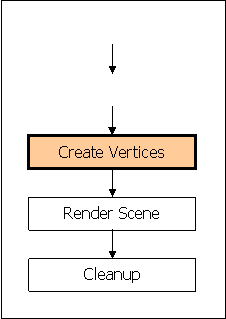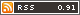## Working With Vertices

In the last tutorial we created our device and started our scene rendering. The only problem was there was nothing to render. Well take care of that in this lesson. We are going to add another step to the diagram that I made for the first tutorial to create three vertices to make a simple triangle. As you can see, we need to create the vertices before we render the scene.Lets take a look at the code we had for the first tutorial because we are going to modify it.

1. Private Dev As Device = Nothing
2. Private Sub frmMain_Load(ByVal sender As System.Object, ByVal e As _
4.   If Init3d() = False Then 'Initialize Direct3D
5.     MsgBox("Could not initialize Direct3D.  This tutorial will exit.")
6.   End If
7.   Me.Show()
8.   Do
9.     Render()
10.     Forms.Application.DoEvents()
11.   Loop
12. End Sub
13. Public Function Init3d() As Boolean
14.   Try
15.     Dim pParams As New PresentParameters()
16.     pParams.Windowed = True
18.     Dev = New Device(0, DeviceType.Hardware, Me, _
19.     CreateFlags.SoftwareVertexProcessing, pParams)
20.     Return (True)
21.   Catch e As DirectXException
22.     Return (False)
23.   End Try
24. End Function
25. Private Sub Render()
26.   Dev.Clear(ClearFlags.Target, System.Drawing.Color.Blue, 1.0F, 0)
27.   Dev.BeginScene()
28.   '=============
29.   Dev.EndScene()
30.   Dev.Present()
31. End Sub

All that were doing here is just initializing the objects (in this case the only object is the device) and then we continually render the scene in a loop. Next we have to add the vertices for our triangle.

First we need to declare an object for our Vertex Buffer.

1. Private VertBuf As vertexBuffer = Nothing

We can put this at the top near the declaration for the device object. Now we can add the vertex info for the triangle.

1. Public Sub MakeVerts()
2.   VertBuf = New VertexBuffer(GetType( _
3.   CustomVertex.TransformedColored), 3, Dev, 0, _
4.   CustomVertex.TransformedColored.Format, Pool.Default)
5.   Dim verts As CustomVertex.TransformedColored() = _
6.   CType(VertBuf.Lock(0, 0), CustomVertex.TransformedColored())
7.
8.   verts(0).X = 150
9.   verts(0).Y = 50
10.   verts(0).Z = 0.5F
11.   verts(0).Rhw = 1
12.   verts(0).Color = System.Drawing.Color.Aqua.ToArgb()
13.   verts(1).X = 250
14.   verts(1).Y = 250
15.   verts(1).Z = 0.5F
16.   verts(1).Rhw = 1
17.   verts(1).Color = System.Drawing.Color.Brown.ToArgb()
18.   verts(2).X = 50
19.   verts(2).Y = 250
20.   verts(2).Z = 0.5F
21.   verts(2).Rhw = 1
22.   verts(2).Color = System.Drawing.Color.LightPink.ToArgb()
23.   VertBuf.Unlock()
24. End Sub

Its time to step through the code.

• Line 2 & 3 Makes a VertexBuffer object. This is the object that well use for the triangle. The parameters are pretty self explanatory. Youre telling it what type of vertices its going to have, how many, the device its going to render to, the VertexBuffer usage, the VertexFormat, and it sets how the memory will be managed wit the MemoryPool.
• Line 5 & 6 Sets verts as a colored vertex that will make the points for the triangle in the VertexBuffer. This line also locks the VertexBuffer. This locks the vertex data and obtains the vertex buffer memory.
• Line 8 - 24 Sets the position and color for each point in the triangle.
• Line 25 Unlocks the VertexBuffer.

Now that we have all of our vertices we can render them.

1. Private Sub Render()
2.   Dev.Clear(ClearFlags.Target, System.Drawing.Color.Blue, 1.0F, 0)
3.   Dev.BeginScene()
4.   '==============
5.   Dev.SetStreamSource(0, VertBuf, 0)
6.   Dev.VertexFormat = CustomVertex.TransformedColored.Format
7.   Dev.DrawPrimitives(PrimitiveType.TriangleStrip, 0, 1)
8.   '==============
9.   Dev.EndScene()
10.   Dev.Present()
11. End Sub

The first new line of code binds a vertex buffer to a device data stream. The second line sets the format of the custom vertices that we made earlier. Lastly, the third line draws the vertices. The primitive is the shape made by the vertices. Making it a TriangleStrip will actually make it look like a triangle. The second parameter sets the starting vertex, and the third sets how many primitives will be made with these vertices. Using Triangle Strips or Triangle Fans is sometimes better than using Triangle Lists because there are less vertices that are duplicated.

There are six different Primitive Types.
1. Point Lists
2. Line Lists
3. Line Strips
4. Triangle Lists
5. Triangle Strips
6. Triangle Fans
You can view the different lists at this MSDN site

Finally we can load the vertices in the Init3d function.

1. Public Function Init3d() As Boolean
2.   Try
3.     Dim pParams As New PresentParameters()
4.
5.     pParams.Windowed = True
7.     Dev = New Device(0, DeviceType.Hardware, Me,  _
8.     CreateFlags.SoftwareVertexProcessing, pParams)
9.
10.     MakeVerts()
11.
12.     Return (True)
13.   Catch e As DirectXException
14.     Return (False)
15.   End Try
16. End Function

You now have a very simple (and useless) triangle! Using this method is fine if you want to make simple polygons. But in order to make a complex mesh, its best to use a 3d modeler program and export the mesh to a file type that you can work with in your application. DirectX has built in functions to work with .X files.

My next tutorial will deal with making a simple box with a texture and adding a colored light for a nice effect.

Tutorial by:

### Jason Zurowski

Date: 2003 Nov 23Printer Friendly